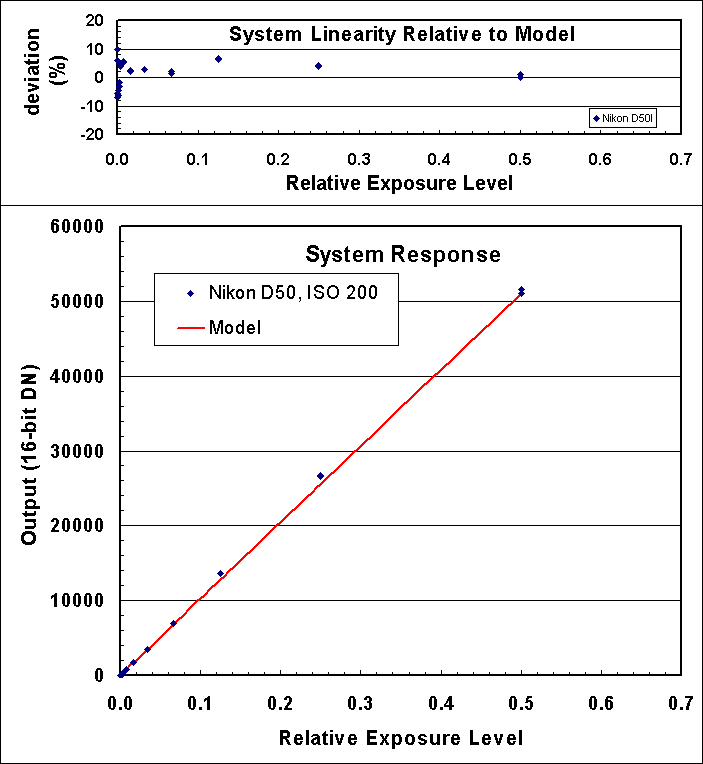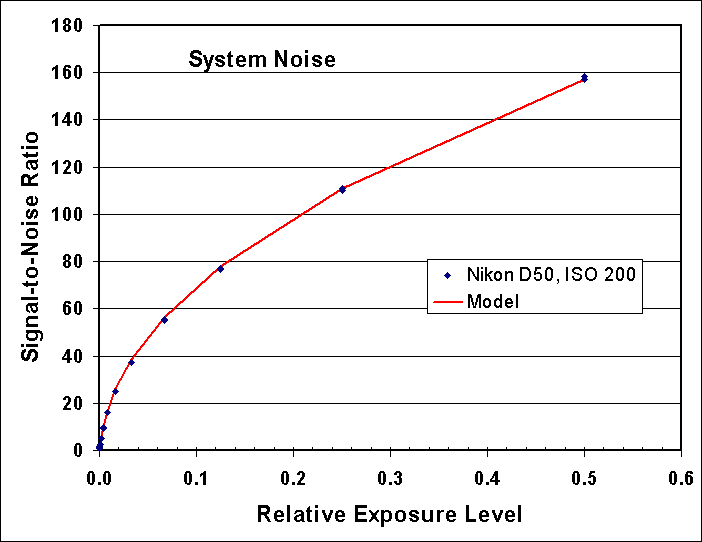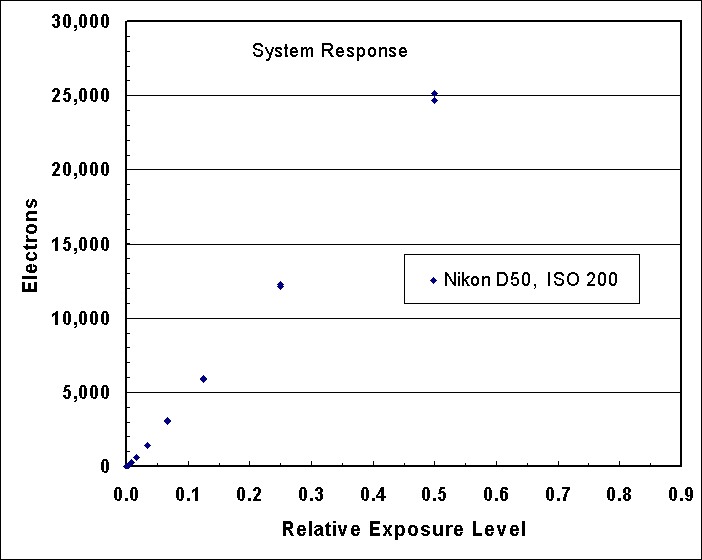# ClarkVision.comHome Galleries Articles Reviews Best Gear Science New About Contact

## The Nikon D50 Digital Camera Review: Sensor Noise, Dynamic Range, and Full Well Analysis

by Roger N. Clark

This page shows an analysis of noise, dynamic range, and full well capacity of a Nikon D50 camera. Raw data were kindly supplied by Duncan Munro who gave permission to use the data for thiS analysis and its publication.

Procedures for performing this analysis are described in: Procedures for Evaluating Digital Camera Noise, Dynamic Range, and Full Well Capacities; Canon 1D Mark II Analysis

The lowest possible noise from a system detecting light is the noise due to Poisson statistics from the random rate of arrival of photons. This is called photon statistics, or photon noise. Noise from the electronics will add to the photon noise. We will see that the Nikon D50 is limited by photon statistics at high signal levels and by electronic noise from reading the sensor (called readout noise) at very low signal levels. In the case of high signal levels, a system that is photon statistics limited enables us to directly measure how many photons the sensor captures, and by increasing the exposure, we can determine how many photons are required to saturate the sensor. That is called the full well capacity. With data on the lowest noise to the highest signal, we can then determine the dynamic range of the sensor.

Results

Results for determining read noise is shown in Table 1. Column B is the gain derived from high signal data. Gains at ISOs higher than 200 are scaled relative to that at ISO 200. Read noise, Column C was determined from exposures with zero light on the sensor. Maximum signal, column D, is 30,490 electrons at ISO 200. Dynamic range, Column E = maximum signal / read noise. Higher ISO reduces the maximum electrons relative to ISO 200 (e.g. ISO 400 sees half the electrons of ISO 200). The maximum signal-to-noise ratio, Column F, is the square root of the number of maximum photons recorded (e.g. square root 30,490 at ISO 200) (read noise is negligible for the high signal case).

Table 1: Nikon D50 Read Noise and Dynamic Range

``` A        B              C          D         E           F
Camera       Apparent
ISO     Gain        Read Noise   Maximum   Dynamic    Maximum
(electrons/    (electrons)  Signal     Range    Signal-to-Noise
12-bit DN)              (electrons)              Ratio

200     7.45          13.4      30,490     2275        175
400     3.72                    15,240                 123
800     1.86           7.47      7,600     1020         87
1600     0.93                     3,800                  62
```

The system linearity is shown in Figure 1, and is very good. System linearity includes the detector linearity, shutter reproducibility, f/stop reproducibility, and light source stability.

The signal-to-noise ratio as a function of intensity is shown in Figure 2. If the sensor is photon noise limited, the trend in this ratio is a square root dependence. If the system is photon noise limited, the signal-to-noise ratio squared directly equals the number of photons collected without needing gain conversions, or computing the slope of a line. The fact that the model matches the data very well indicates there are no other sources of noise contributing to the data at any significant level. Thus, for high signal levels, the Nikon D50 is photon noise limited. This means further improvements in electronics will not improve the signal-to-noise ratio for high signal levels. If the quantum efficiency were improved with a different sensor, that would certainly improve the signal-to-noise ratio.Figure 1. The Nikon D50 shows a very linear trend with signal. Variations in signal level include repeatability of the shutter and aperture, as well as the light source. The model is the average high signal DN scaled according to exposure time and subtract read noise.Figure 2. Signal-to-noise ratio observed and predicted. The prediction model uses photon statistics and read noise. Model is average DN scaled to electrons plus read noise. For this case, ISO 200, the gain is 7.45 electrons/DN, and the read noise is 13.4 electrons.Figure 3. The electrons converted by the Nikon D50 sensor are shown relative to exposure level. The sensor saturates at about 30,490 electrons, but is quite linear below that level.

Dark Current and Related Noise

Another noise component is due to dark current, which will increase with exposure time. Dark current and its noise is also a function of the temperature of the sensor and associated electronics.

The noise model is:

N = (P + r2 + t2)1/2, (eqn 1)

Where N = total noise in electrons, P = number of photons, r = read noise in electrons, and t = thermal noise in electrons. Noise from a stream of photons, the light we all see and image with our cameras, is the square root of the number of photons, so that is why the P in equation 1 is not squared (sqrt(P)2 = P).

Conclusions

The data shown here for the Nikon D50 shows that the camera is operating and near perfect levels for the sensor. This means that for high signals, noise is dominated by photon statistics. Noise at low signal levels can be improved, but is quite good. To achieve higher signal-to-noise ratio images, a higher quantum efficiency sensor with a larger full well would be needed. Without increasing the full well capacity, the maximum signal-to-noise ratio would not improve, but the signal-to-noise ratio at ISO 200 could be achieved at higher ISOs if the quantum efficiency were improved. Improvements in quantum efficiency of about 3 are possible (Reference 2).

References

Notes:

DN is "Data Number." That is the number in the file for each pixel. I'm quoting the luminance level (although red, green and blue are almost the same in the cases I cited).

16-bit signed integer: -32768 to +32767

16-bit unsigned integer: 0 to 65535

Photoshop uses signed integers, but the 16-bit tiff is unsigned integer (correctly read by ImagesPlus).

 Home Galleries Articles Reviews Best Gear Science New About Contact

http://www.clarkvision.com/reviews/evaluation-nikon-d50

First published March 5, 2006.
Last updated March 6, 2006